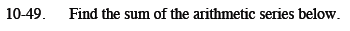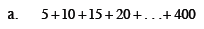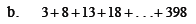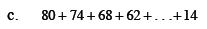Home > CCA2 > Chapter 10 > Lesson 10.1.3 > Problem10-49

10-49.
1. Find the sum of the arithmetic series below. Homework Help ✎

1. 5 + 10 + 15 + 20 + … + 400

2. 3 + 8 + 13 + 18 + … + 398

3. 80 + 74 + 68 + 62 + … + 14Find the sequence that describes the series.

t(n) = 5n

Find which term is 400.

(400) = 5n
80 = n

Find the sum using the formula from the Math Notes box.

$S(80)=\frac{80(5+400)}{2}$

16200See part (a).

16040See part (a).